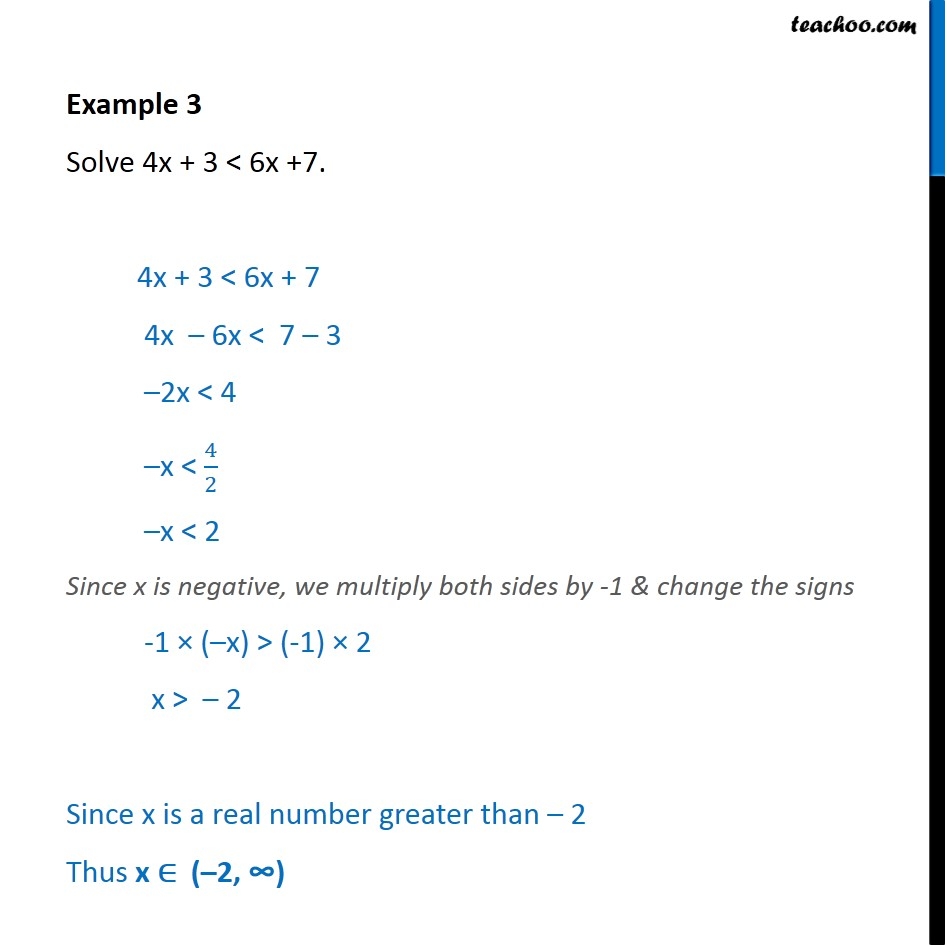1. Chapter 6 Class 11 Linear Inequalities (Term 2)
2. Serial order wise
3. Examples

Transcript

Example 3 Solve 4x + 3 < 6x +7. 4x + 3 < 6x + 7 4x 6x < 7 3 2x < 4 x < 4 2 x < 2 Since x is negative, we multiply both sides by -1 & change the signs -1 ( x) > (-1) 2 x > 2 Since x is a real number greater than 2 Thus x ( 2,

Examples

Chapter 6 Class 11 Linear Inequalities (Term 2)
Serial order wise